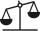# Equation 20

In given equation:

8/9-4/5=2/9+x,

find x

Result

x =  -0.133

#### Solution:

8/9-4/5=2/9+x

45x = -6

x = -215 ≈ -0.133333

Calculated by our simple equation calculator.

Leave us a comment of example and its solution (i.e. if it is still somewhat unclear...):

Showing 0 comments:Be the first to comment!#### To solve this example are needed these knowledge from mathematics:

Need help calculate sum, simplify or multiply fractions? Try our fraction calculator. Do you have a linear equation or system of equations and looking for its solution? Or do you have quadratic equation?

## Next similar examples:

1. EquationFind x: x + 1/2 = 1/3
2. Simple equation 5Solve equation with fractions: X × 3/8 = 1/2
3. Equation 15Solve equation with variables on both sides:
4. TreesFrom the total number of trees in the orchard, there are two-fifths pearls and apples are three eighty. The rest of the trees are 9 ceremonial. How many trees are in the set?
5. Brick weightHalf the weight of a brick plus 20 pounds is equal to 1/3 the weight of the brick plus 30 pounds. How much does the brick weigh?
6. Trees3/5 trees are apples, cherries are 1/3. 5 trees are pear. How many is the total number of trees?
7. FruitsAmy bought a basket of fruits 1/5 of them were apples,1/4 were oranges, and the rest were 33 bananas. How many fruits did she buy in all?
8. NumberI think the number. If I add to its third seven I get same as when to its quarter add 8. Which is the number?
9. BrickIsosceles scale has on one side all brick and second weight 1 kg and 1/4 of brick. The balance is in equilibrium. What is the weight of a brick?
10. Cakes1/3 poppy cake, 1/3 apple, 15 pieces of cheese. How many are totally cakes?
11. Forest nurseryIn the forest nursery after winter, they found that 1/10 stems died out of them. For them, they land 193 new spruces. How many spruces are in the forest nursery?
12. Mixed2improperWrite the mixed number as an improper fraction. 166 2/3
13. Fraction and a decimalWrite as a fraction and a decimal. One and two plus three and five hundredths
14. Fraction to decimalWrite the fraction 3/22 as a decimal.
15. In fractionsAn ant climbs 2/5 of the pole on the first hour and climbs 1/4 of the pole on the next hour. What part of the pole does the ant climb in two hours?
16. ZdeněkZdeněk picked up 15 l of water from a 100-liter full-water barrel. Write a fraction of what part of Zdeněk's water he picked.
17. Lengths of the poolMiguel swam 6 lengths of the pool. Mat swam 3 times as far as Miguel. Lionel swam 1/3 as far as Miguel. How many lengths did mat swim?